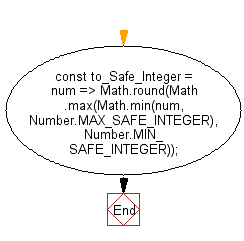# JavaScript: Convert a value to a safe integer

## JavaScript fundamental (ES6 Syntax): Exercise-29 with Solution

Write a JavaScript program to convert a value to a safe integer.

• Use Math.max() and Math.min() to find the closest safe value.
• Use Math.round() to convert to an integer.

Sample Solution:

JavaScript Code:

``````//#Source https://bit.ly/2neWfJ2
const to_Safe_Integer = num =>
Math.round(Math.max(Math.min(num, Number.MAX_SAFE_INTEGER), Number.MIN_SAFE_INTEGER));
console.log(to_Safe_Integer('5.2'));
console.log(to_Safe_Integer('5.52'));
console.log(to_Safe_Integer(Infinity));
```
```

Sample Output:

```5
6
9007199254740991
```

Flowchart:Live Demo:

See the Pen javascript-basic-exercise-1-29 by w3resource (@w3resource) on CodePen.

Improve this sample solution and post your code through Disqus

What is the difficulty level of this exercise?

Test your Programming skills with w3resource's quiz.

﻿